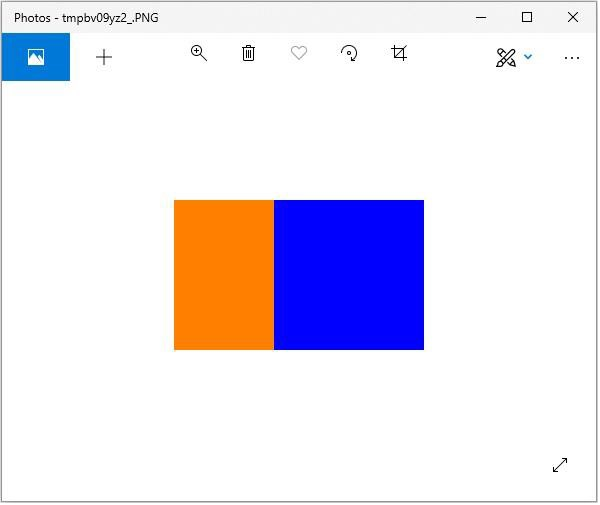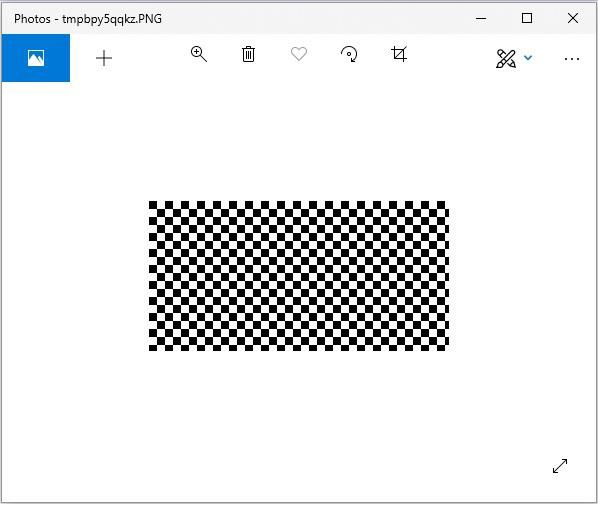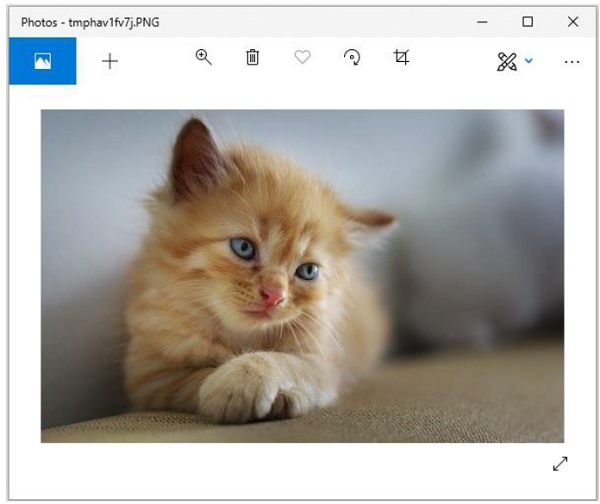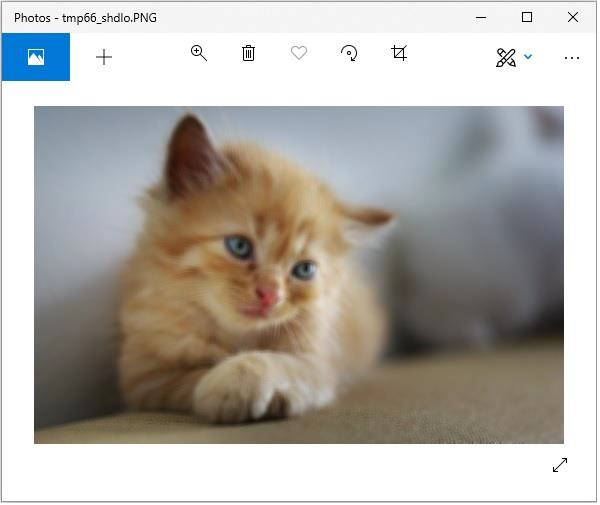# Python Pillow - M L with Numpy

In this chapter, we use numpy to store and manipulate image data using python imaging library – “pillow”.

Before proceeding with this chapter open command prompt in administrator mode and execute the following command in it to install numpy −

```pip install numpy
```

Note − This works only if you have PIP installed and updated.

## Creating image from Numpy Array

Creating an RGB image using PIL and save it as a jpg file. In the following example we will −

• Create a 150 by 250-pixel array.

• Fill left half of the array with orange.

• Fill right half of the array with blue.

```from PIL import Image
import numpy as np

arr = np.zeros([150, 250, 3], dtype=np.uint8)

arr[:,:100] = [255, 128, 0]

arr[:,100:] = [0, 0, 255]

img = Image.fromarray(arr)

img.show()

img.save("RGB_image.jpg")
```

### Output## Creating greyscale images

Creating greyscale images is slightly different from creating an RGB image. We can use the 2-dimensional array to create a greyscale image.

```from PIL import Image
import numpy as np

arr = np.zeros([150,300], dtype=np.uint8)

#Set grey value to black or white depending on x position
for x in range(300):
for y in range(150):
if (x % 16) // 8 == (y % 16)//8:
arr[y, x] = 0
else:
arr[y, x] = 255
img = Image.fromarray(arr)

img.show()

img.save('greyscale.jpg')
```

### Output## Creating numpy array from an Image

You can convert PIL image to numpy array and vice versa. A small program to demonstrate the same is stated below.

### Example

```#Import required libraries
from PIL import Image
from numpy import array

#Open Image & create image object
img = Image.open('beach1.jpg')

#Show actual image
img.show()

#Convert an image to numpy array
img2arr = array(img)

#Print the array
print(img2arr)

#Convert numpy array back to image
arr2im = Image.fromarray(img2arr)

#Display image
arr2im.show()

#Save the image generated from an array
arr2im.save("array2Image.jpg")
```

### Output

If you save the above program as Example.py and execute −

• It displays the original image.

• Converts the array back into image and displays it.

• Since we have used show() method the images are displayed using the default PNG display utility, as follows.

```[[[ 0 101 120]
[ 3 108 127]
[ 1 107 123]
...
...
[[ 38 59 60]
[ 37 58 59]
[ 36 57 58]
...
[ 74 65 60]
[ 59 48 42]
[ 66 53 47]]
[[ 40 61 62]
[ 38 59 60]
[ 37 58 59]
...
[ 75 66 61]
[ 72 61 55]
[ 61 48 42]]
[[ 40 61 62]
[ 34 55 56]
[ 38 59 60]
...
[ 82 73 68]
[ 72 61 55]
[ 63 52 46]]]
```

Original ImageImage constructed from the array